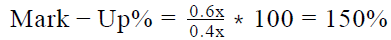Courses

# A shopkeeper offers two successive discounts of 20% each on a sweater and still makes a profit of 60%. By what percentage didthe shopkeeper mark-up the price of the sweater?a)60%b)100%c)150%d)200%e)250%Correct answer is option 'C'. Can you explain this answer? Related Test: Test: Sale And Purchase- 2

## GMAT Question

By MALI MRUNALI YASHWANT · Mar 23, 2020 ·GMAT
Harmanjot Singh answered Apr 06, 2020
Given:
• Successive discounts (d and d ) offered by the shopkeeper on a sweater
• d = 20%
• d = 20%
• Profit % of the shopkeeper = 60%
To Find: Mark-up% on the sweater?
Approach:
1.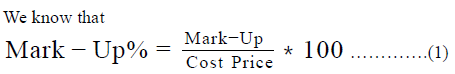• So, in order to know the MarkUp %, we need to know either the ratio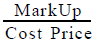or the values of Markup (the amount of markup) and the Cost Price. Since we are not given the dollar values of anything (like cost price, selling price, marked price, discount or profit), we will not be able to find the dollar values of MarkUp and Cost Price. So, we should try to find the ratio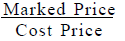2.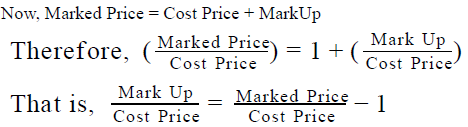• So, in order to find the ratio we need to find the ratio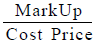we need to fid the ratio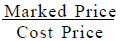3. We are given the discounts availed on the sweater. Using these discounts, we will be able to find the ratio of Marked Price and Selling Price of the sweater.
• Let’s assume the Marked Price to be x.
• So, Marked Price after applying discount d1 = x – d1 % of x
• Now, Marked Price after applying discount d2 = (x – d1% of x) – d2 % of (x – d1 % of x)
So, the selling price of the sweater = (x – d1 % of x) – d2 % of (x  – d1 % of x)
• So,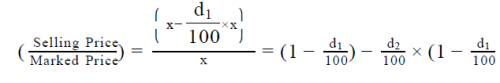We are also given the profit % on the sweater. Using this, we will be able to find the ratio of Selling Price and Cost Price of the
sweater
Selling Price = Cost Price + Profit
Also, we know that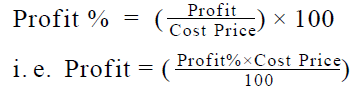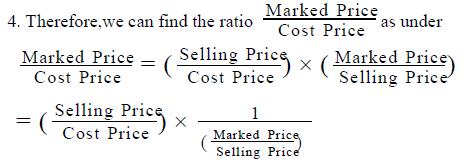Working out: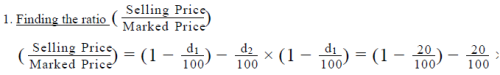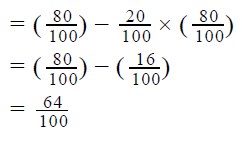2. Calculating the Cost Price
a. Selling Price = 0.64x
b. Profit% = 60%
c. So, we can write 0.64x = CP + 60% of CP, which gives us CP = 0.4x

3. Calculating Mark-Up%
a. Hence, the price of sweater was marked up by = x – 0.4x = 0.6x. So, using (1), the mark-up5 can be given by